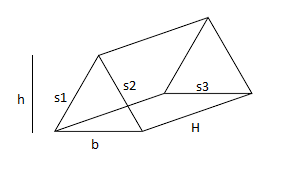Related Articles

# Program to calculate the Surface Area of a Triangular Prism

• Last Updated : 18 Mar, 2021

In mathematics, a triangular prism is a three-dimensional solid shape with two identical ends connected by equal parallel lines, and have 5 faces, 9 edges, and 6 vertices.where “b” is the length of the base, “h” is the height of the triangle, “s1, s2, s3” are the respective length of each side of the triangle, and H is the height of the prism (which is also the length of the rectangle).
Given the base, the height of the triangle, height of prism and the length of each side of triangle base and the task is to calculate the surface area of the triangular prism.
Examples:

Input: b = 3, h = 4, s1 = 3, s2 = 6, s3 = 6, Ht = 8
Output: The area of triangular prism is 132.000000
Input: b = 2, h = 3, s1 = 4, s2 = 5, s3 = 6, Ht = 8
Output: The area of triangular prism is 126.000000

Formula for calculating the surface area:
As stated above, the prism contains two triangles of the area (1/2)*(b)*(h) and three rectangles of the area H*s1, H*s2 and H*s3
Now after adding all the terms we get the total surface area:

`SA = b * h + (s1 + s2 + s3 ) * H`

## C++

 `// C++ Program to calculate the``// Surface area of a triangular prism``#include ``using` `namespace` `std;` `// Function for calculating the area``void` `Calculate_area()``{``    ``// Initialization``    ``float` `b = 3, h = 4, s1 = 3, s2 = 6;``    ``float` `s3 = 6, Ht = 8, SA;` `    ``// Formula for calculating the area``    ``SA = b * h + (s1 + s2 + s3) * Ht;` `    ``// Displaying the area``    ``cout << ``"The area of triangular prism is : "` `<< SA;``}` `// Driver code``int` `main()``{``    ``// Function calling``    ``Calculate_area();` `    ``return` `0;``}`

## C

 `// C Program to calculate the``// Surface area of a triangular prism``#include ` `// Function for calculating the area``void` `Calculate_area()``{``    ``// Initialization``    ``float` `b = 3, h = 4, s1 = 3, s2 = 6;``    ``float` `s3 = 6, Ht = 8, SA;` `    ``// Formula for calculating the area``    ``SA = b * h + (s1 + s2 + s3) * Ht;` `    ``// Displaying the output``    ``printf``(``"The area of triangular prism is : %f"``, SA);``}` `// Driver code``int` `main()``{``    ``// Function calling``    ``Calculate_area();` `    ``return` `0;``}`

## Java

 `// Java Program to calculate the``// Surface area of a triangular prism` `import` `java.util.Scanner;``public` `class` `Prism {` `    ``public` `static` `void` `Calculate_area()``    ``{``        ``// Initialization``        ``double` `b = ``3``, h = ``4``, s1 = ``3``, s2 = ``6``;``        ``double` `s3 = ``6``, Ht = ``8``, SA;` `        ``// Formula for calculating the area``        ``SA = b * h + (s1 + s2 + s3) * Ht;` `        ``// Displaying the area``        ``System.out.printf(``"The area of triangular prism is : %f"``, SA);``    ``}``    ``public` `static` `void` `main(String[] args)``    ``{``        ``Calculate_area();``    ``}``}``// This code is contributed by Nishant Tanwar`

## Python3

 `# Python3 Program to calculate the``# Surface area of a triangular prism` `# Function for calculating the area``def` `Calculate_area():``    ` `    ``# Initialization``    ``b ``=` `3``    ``h ``=` `4``    ``s1 ``=` `3``    ``s2 ``=` `6``    ``s3 ``=` `6``    ``Ht ``=` `8` `    ``# Formula for calculating the area``    ``SA ``=` `b ``*` `h ``+` `(s1 ``+` `s2 ``+` `s3) ``*` `Ht` `    ``# Displaying the area``    ``print` `(``"The area of triangular prism is :"``,SA)` `# Driver code``if` `__name__ ``=``=` `'__main__'``:``    ``# Function calling``    ``Calculate_area()` `# This code is contributed by``# Surendra_Gangwar`

## C#

 `// C# Program to calculate the``// Surface area of a triangular prism``using` `System;``public` `class` `Prism {`` ` `    ``static` `void` `Calculate_area()``    ``{``        ``// Initialization``        ``double` `b = 3, h = 4, s1 = 3, s2 = 6;``        ``double` `s3 = 6, Ht = 8, SA;`` ` `        ``// Formula for calculating the area``        ``SA = b * h + (s1 + s2 + s3) * Ht;`` ` `        ``// Displaying the area``        ``Console.WriteLine(``"The area of triangular prism is : "` `+ SA);``    ``}``    ``static` `public` `void` `Main(String[] args)``    ``{``        ``Calculate_area();``    ``}``}`

## PHP

 ``

## Javascript

 ``
Output
`The area of triangular prism is : 132`

Attention reader! Don’t stop learning now. Get hold of all the important mathematical concepts for competitive programming with the Essential Maths for CP Course at a student-friendly price. To complete your preparation from learning a language to DS Algo and many more,  please refer Complete Interview Preparation Course.

My Personal Notes arrow_drop_up